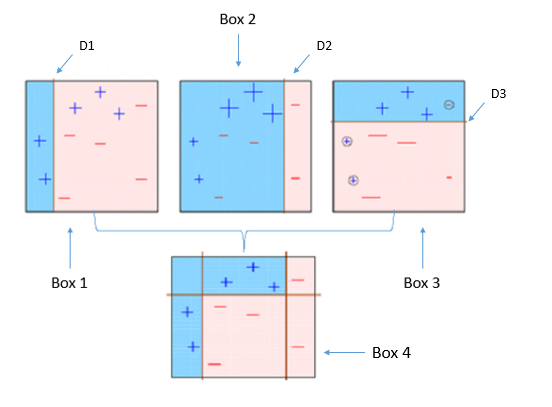Class discussion

This is a diagram explaining boosting. The three tree models in the top row are combined to give the boosted model in box 4. Come up with a some words and sentences, together, to explain the process.How would a single tree with multiple splits fit this data? What is different about the two approaches?

Activities

• Sign up for a kaggle account.
• Upload s set of predictions for the tennis data challenge.

Do it yourself

This exercise is based on the lab material in chapter 8 of the textbook, and exercise 11. Solutions to the textbook exercise can be found at https://blog.princehonest.com/stat-learning/ch8/11.html.

1. Use the Caravan data from the ISLR package. Read the data description.
1. Compute the proportion of caravans purchased to not purchased. Is this a balanced class data set? What problem might be encountered in assessing the accuracy of the model as a consequence?
2. Convert the response variable from a factor to an integer variable, where 1 indicates that the person purchased a caravan.
3. Break the data into 2/3 training and test set, ensuring that the same ratio of the response variable is achieved in both sets. Check that your sampling has produced this.
4. The solution code on the unofficial solution web site:
library(ISLR)
train = 1:1000
Caravan$Purchase = ifelse(Caravan$Purchase == "Yes", 1, 0)
Caravan.train = Caravan[train, ]
Caravan.test = Caravan[-train, ]

would use just the first 1000 cases for the training set. What is wrong about doing this?

1. Here we will fit a boosted tree model, using the gbm package.
1. Use 1000 trees, and a shrinkage value of 0.01.
2. Make a plot of the oob improvement against iteration number. What does this suggest about the number of iterations needed? Why do you think the oob improvement value varies so much, and can also be negative?
3. Compute the error for the test set, and for each class. Consider a proportion 0.2 or greater to indicate that the customer will purchase a caravan.
4. What are the 6 most important variables? Make a plot of each to examine the relationship between these variables and the response. Explain what you learn from these plots.
1. Here we will fit a random forest model, using the randomForest package.
1. Use 1000 trees, using a numeric response so that predictions will be a number between 0-1, and set importance=TRUE. (Ignore the warning about not having enough distinct values to use regression.)
2. Compute the error for the test set, and for each class. Consider a proportion 0.2 or greater to indicate that the customer will purchase a caravan.
3. What are the 6 most important variables? Make a plot of any that are different from those chosen by gbm. How does the set of variables compare with those chosen by gbm.
1. Here we will fit a gradient boosted model, using the xgboost package.
1. Read the description of the XGBoost technique at https://www.hackerearth.com/practice/machine-learning/machine-learning-algorithms/beginners-tutorial-on-xgboost-parameter-tuning-r/tutorial/, or other sources. Explain how this algorithm might differ from earlier boosted tree algorithms.
2. Tune the model fit to determine how many iterations to make. Then fit the model, using the parameter set provided.
3. Compute the error for the test set, and for each class. Consider a proportion 0.2 or greater to indicate that the customer will purchase a caravan.
4. Compute the variable importance. What are the 6 most important variables? Make a plot of any that are different from those chosen by gbm or randomForest. How does the set of variables compare with the other two methods.
1. Compare and summarise the results of the three model fits.